Computer Science

# Practice with variables

These problems will help you practice with variables. Remember that we can define functions with two parameters:

``````def go(bit, color):
""" Given a bit and a color, move and paint that color until blocked """
while bit.front_clear():
bit.move()
bit.paint(color)``````

and then call them by passing in the parameters:

``go(bit, 'green')``

We can also use variables to hold values:

``my_color = 'red'``

and then pass those variables as parameters:

``````my_color = 'red'
go(bit, my_color)``````

Finally, we can get the current color and store it in a variable:

``found_color = bit.get_color()``

## Coloring columns

In this problem, Bit needs to find the color for each column in the world: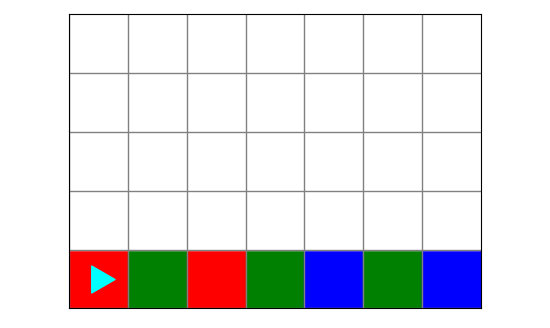and then fill these colors in the column above: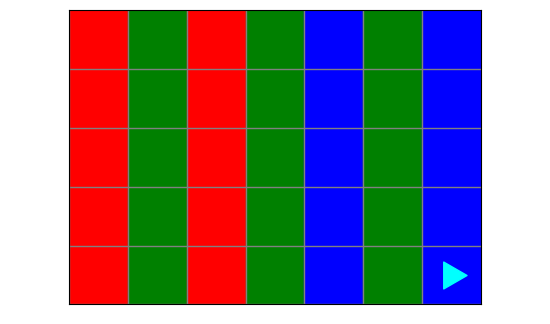### Planning

How would you solve this problem? Draw out your idea.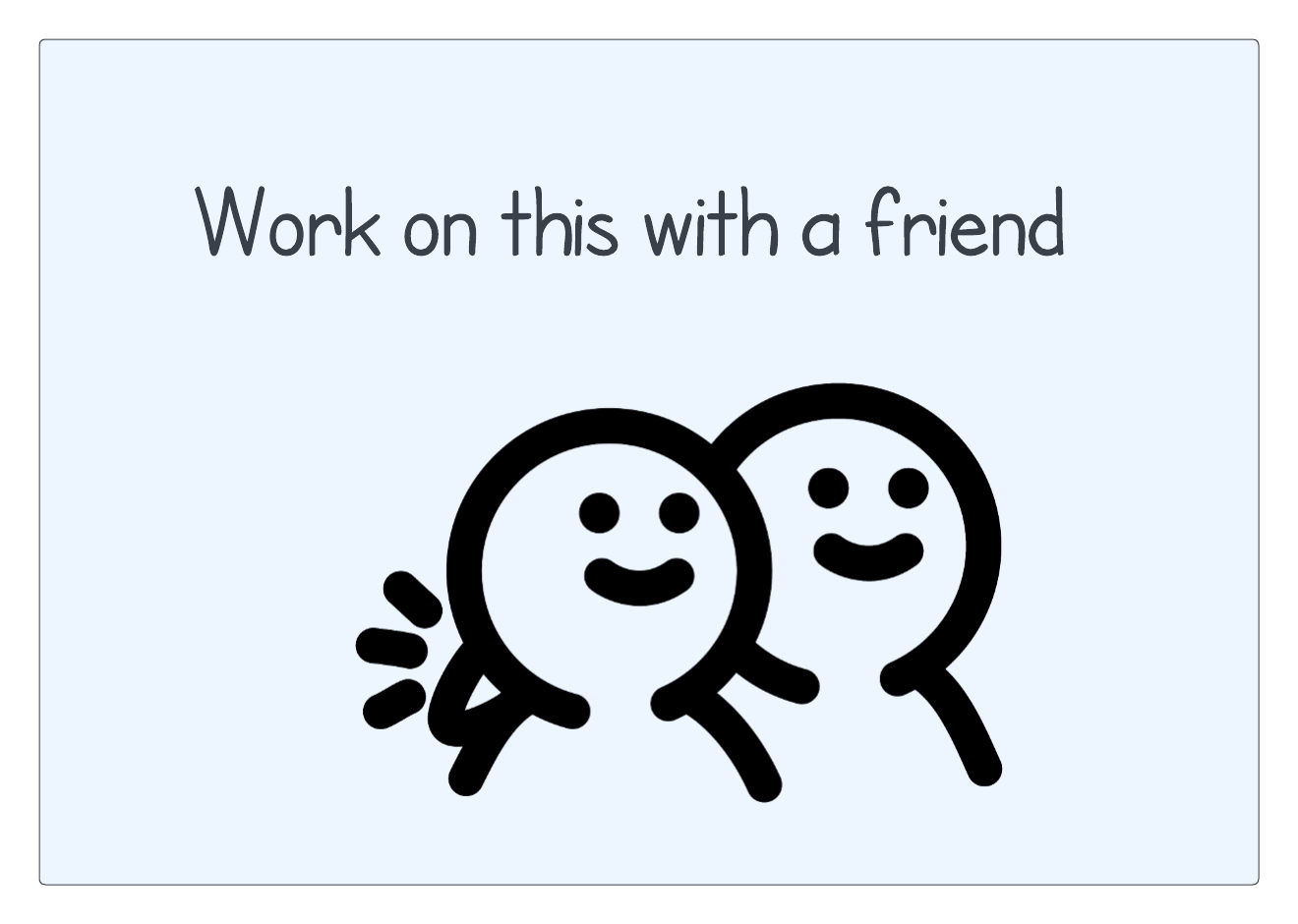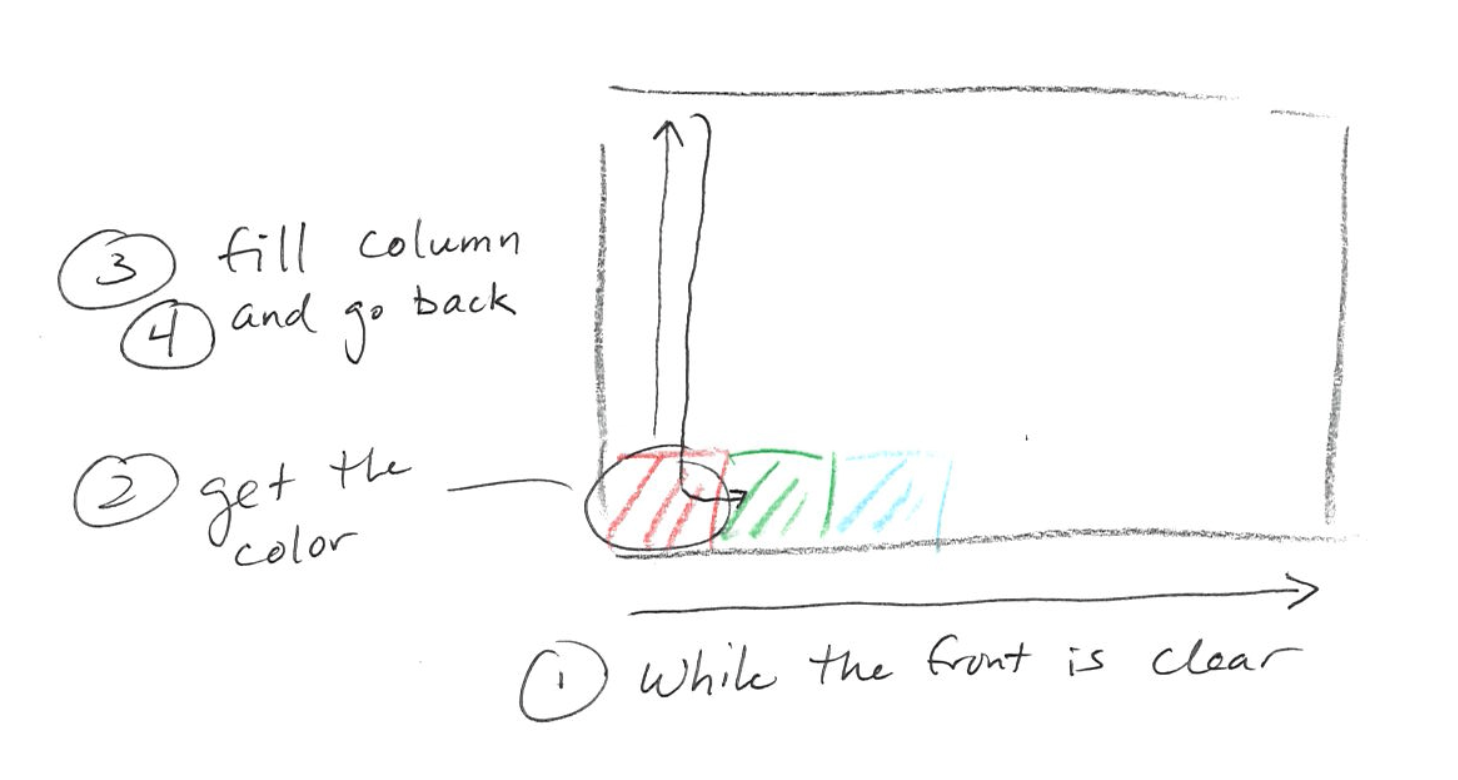• while the front is clear
• get the current color
• fill the column
• go back

### Coding

You can see starter code for this problem in `fill_colorful.py`:

``````from byubit import Bit

@Bit.worlds('colors')
def fill_colorful(bit):
# Write code here
pass

if __name__ == '__main__':
fill_colorful(Bit.new_bit)``````

Let’s put the main loop in `fill_colorful()`:

``````def fill_colorful(bit):
while bit.front_clear():
color = bit.get_color()
fill_column(bit, color)
go_back(bit)
bit.move()``````

Then we can write `fill_column()`:

``````def fill_column(bit, color):
"""
Fills a column with a given color. Bit starts facing right and ends
facing up at the top of the column
"""
bit.left()
while bit.front_clear():
bit.move()
bit.paint(color)``````

and we can fill in `pass` for `go_back()`:

``````def go_back(bit):
pass``````

Let’s see if we got the first part of this problem solved by running the code: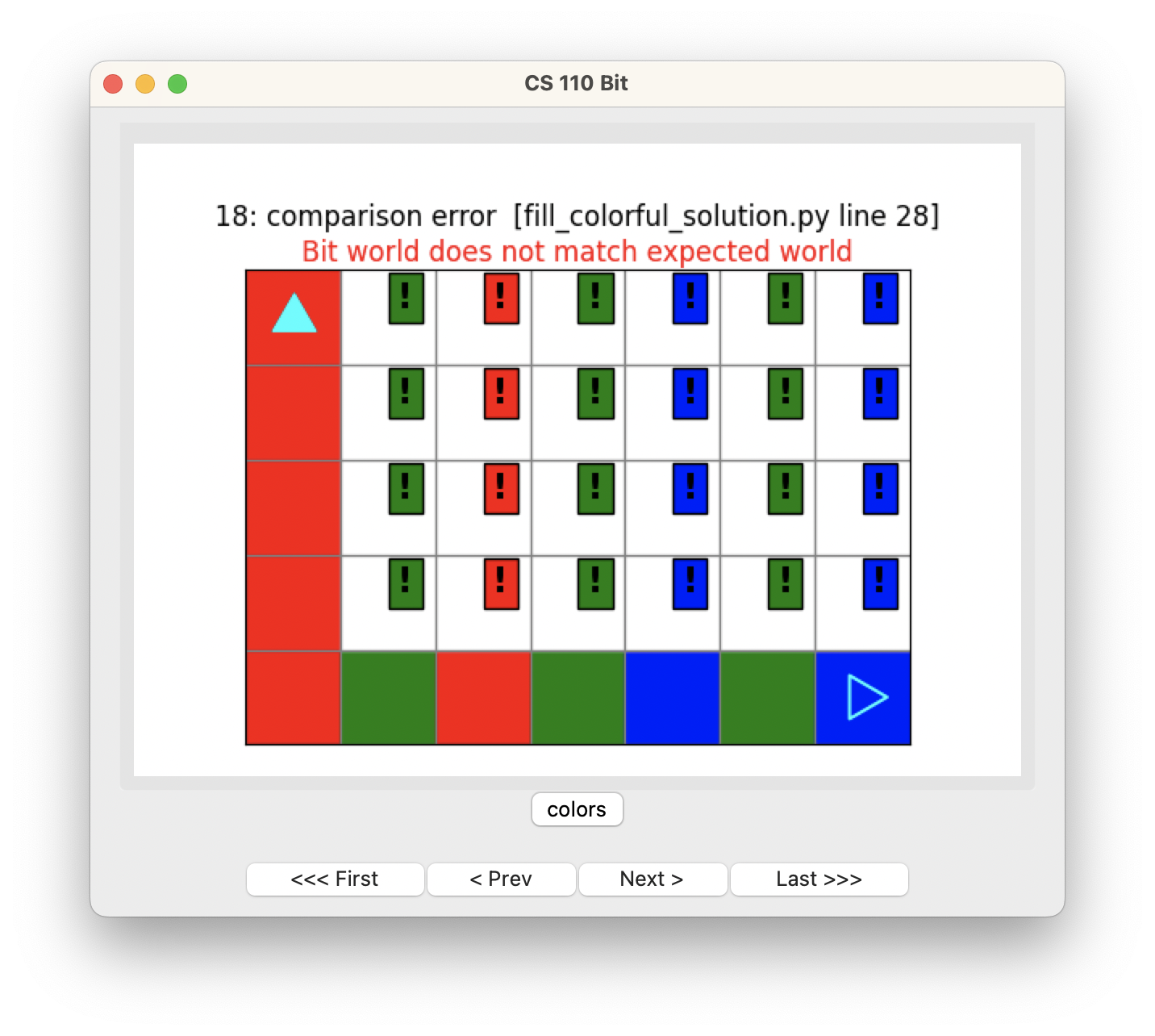Pretty good!

Now we can fill in `go_back()`:

``````def go_back(bit):
"""
Go back to the bottom and turn to the right.
Bit starts by facing up at the top of the column.
Bit ends by facing right at the bottom of the column.
"""
# turn around
bit.right()
bit.right()
# go back
while bit.front_clear():
bit.move()
# turn
bit.left()``````

Let’s run this code to see if we got the logic right to go back: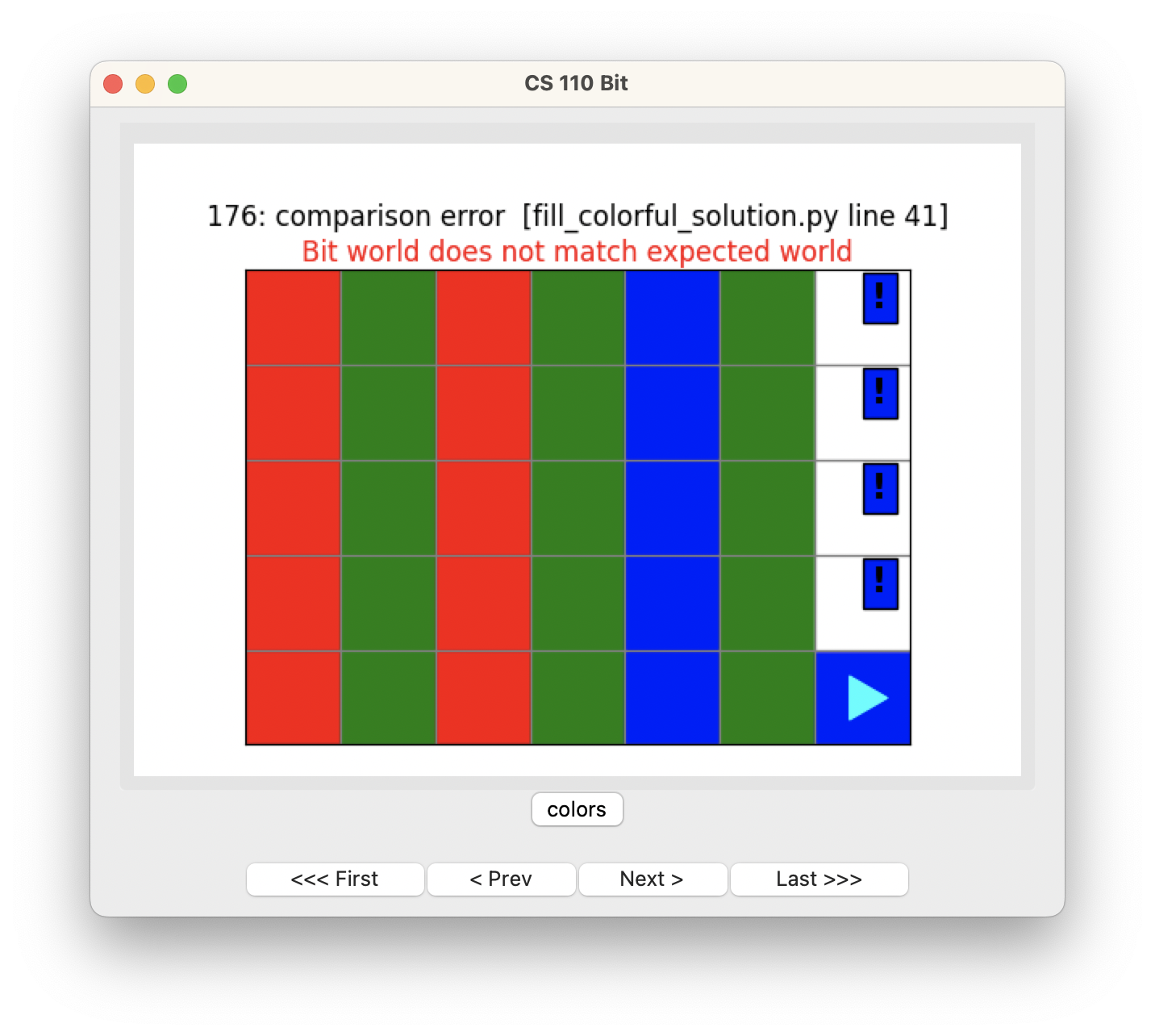Nice. So all we need is to do the last column. We can do this by adding a few lines at the end of our main loop:

``````def fill_colorful(bit):
while bit.front_clear():
color = bit.get_color()
fill_column(bit, color)
go_back(bit)
bit.move()

# do the last column
color = bit.get_color()
fill_column(bit, color)
go_back(bit)``````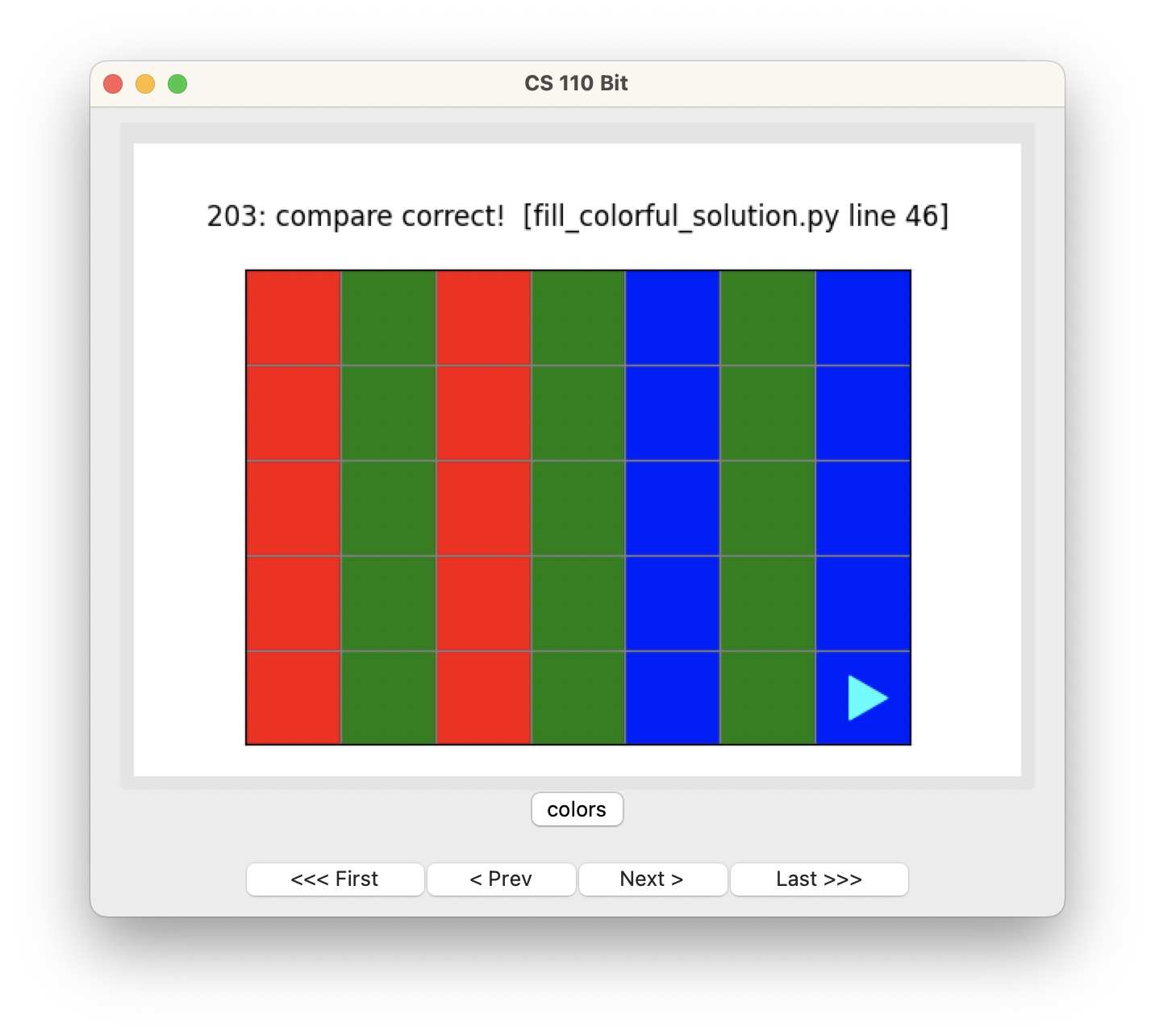Great!

### A few notes

Once you start decomposing problems into multiple functions, you will see that there are lots of ways to solve problems. In this case, we could have put `color = bit.get_color()` into `fill_column()`. We also could have written a function `fill_column_and_go_back()` that called `fill_column()` and `go_back()`.

Ultimately, how you choose to break down the problem depends on what you think makes your code easiest to read. Maybe this is easier:

``````def fill_colorful(bit):
while bit.front_clear():
fill_column_and_go_back(bit)
bit.move()

# do the last column
fill_column_and_go_back(bit)``````

## Coloring Ts

In this problem, Bit starts in a world that provides colored squares: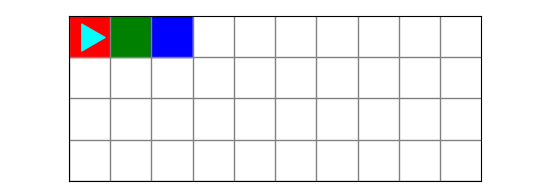Bit’s job is to get these colors, store them, erase them, and then draw three letter `T`s with these colors: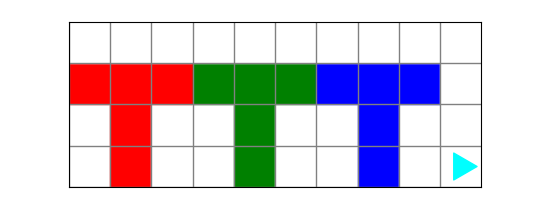The colors can be different! There is a second world that starts like this: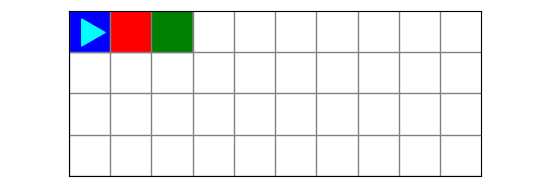### Planning

How would you solve this problem? Draw out your idea.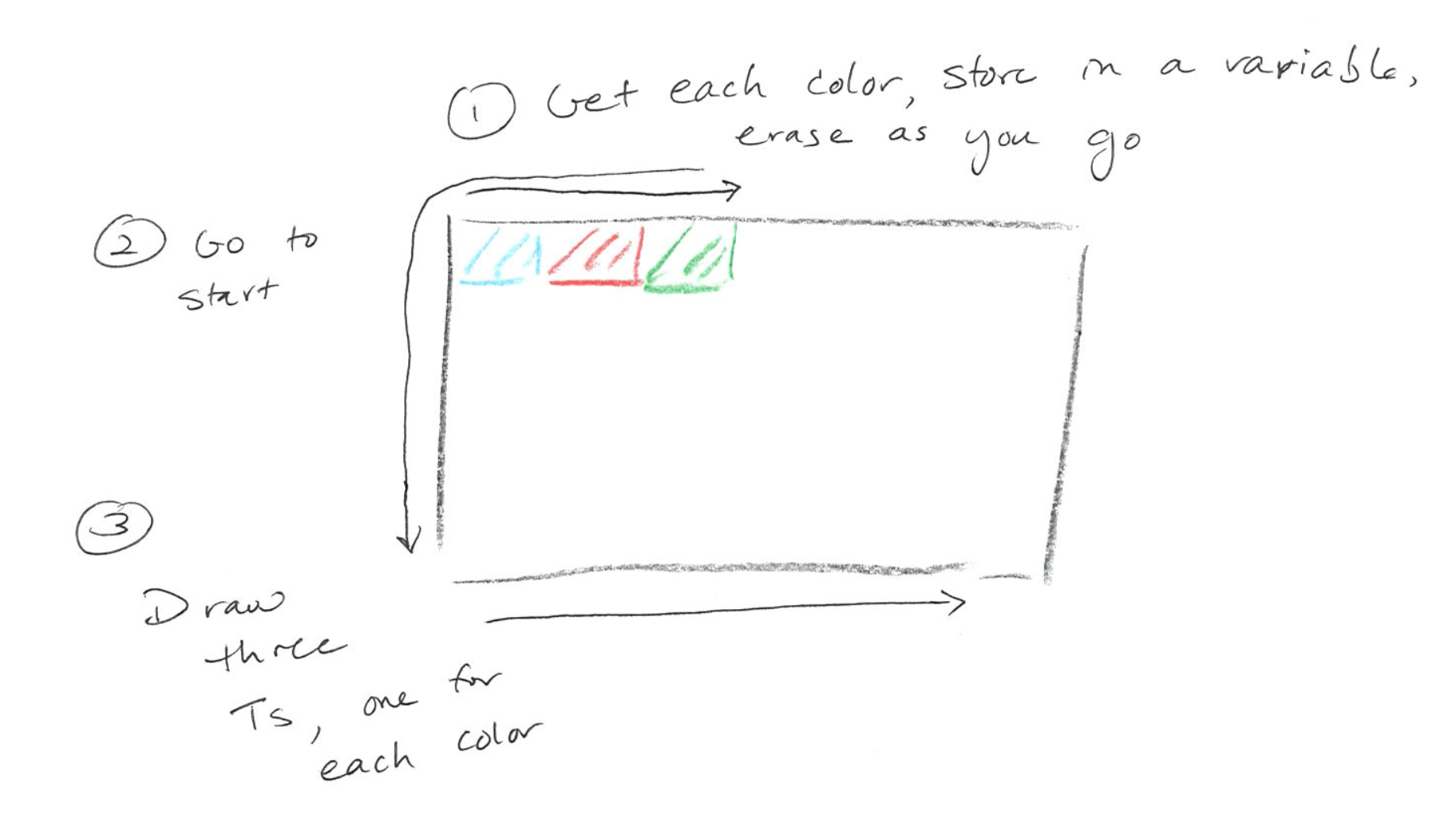• get each color, store it in a variable, erasing as you go
• go to the start
• draw three Ts, one for each color

### Coding

We have given you starter code in `color_tt.py` that provides much of the code you need:

``````from byubit import Bit

def go(bit):
"""Go until blocked in front."""
while bit.front_clear():
bit.move()

def go_to_start(bit):
"""Bit starts anywhere on the board and ends in the bottom left corner facing right."""
bit.left()
bit.left()
go(bit)
bit.left()
go(bit)
bit.left()

def paint_t(bit, color):
"""Paint a T. Start in the bottom left of the 3x3 box. End just outside the bottom right of the 3x3 box."""
bit.move()
bit.left()
bit.paint(color)
bit.move()
bit.paint(color)
bit.move()
bit.left()
bit.move()
bit.left()
bit.left()
bit.paint(color)
bit.move()
bit.paint(color)
bit.move()
bit.paint(color)
bit.right()
bit.move()
bit.move()
bit.left()
bit.move()
bit.snapshot('T painted')

@Bit.worlds('color_tt', 'color_tt2')
def run(bit):
pass

if __name__ == '__main__':
run(Bit.new_bit)``````

All you need is to fill in the piece of this that collects and erases the colors, then calls the other functions. We can get one of the colors like this:

``````def run(bit):
# Get colors
first_color = bit.get_color()
bit.erase()
bit.move()``````

Now just repeat to get all three:

``````def run(bit):
# Get colors
first_color = bit.get_color()
bit.erase()
bit.move()

second_color = bit.get_color()
bit.erase()
bit.move()

third_color = bit.get_color()
bit.erase()
bit.move()``````

Then call the other functions we provided:

``````def run(bit):
# Get colors
first_color = bit.get_color()
bit.erase()
bit.move()

second_color = bit.get_color()
bit.erase()
bit.move()

third_color = bit.get_color()
bit.erase()
bit.move()
bit.snapshot('Got colors')

go_to_start(bit)
bit.snapshot('At start')

paint_t(bit, first_color)
paint_t(bit, second_color)
paint_t(bit, third_color)``````

We have added `bit.snapshot()` so that you can easily step through the code and see how it works.